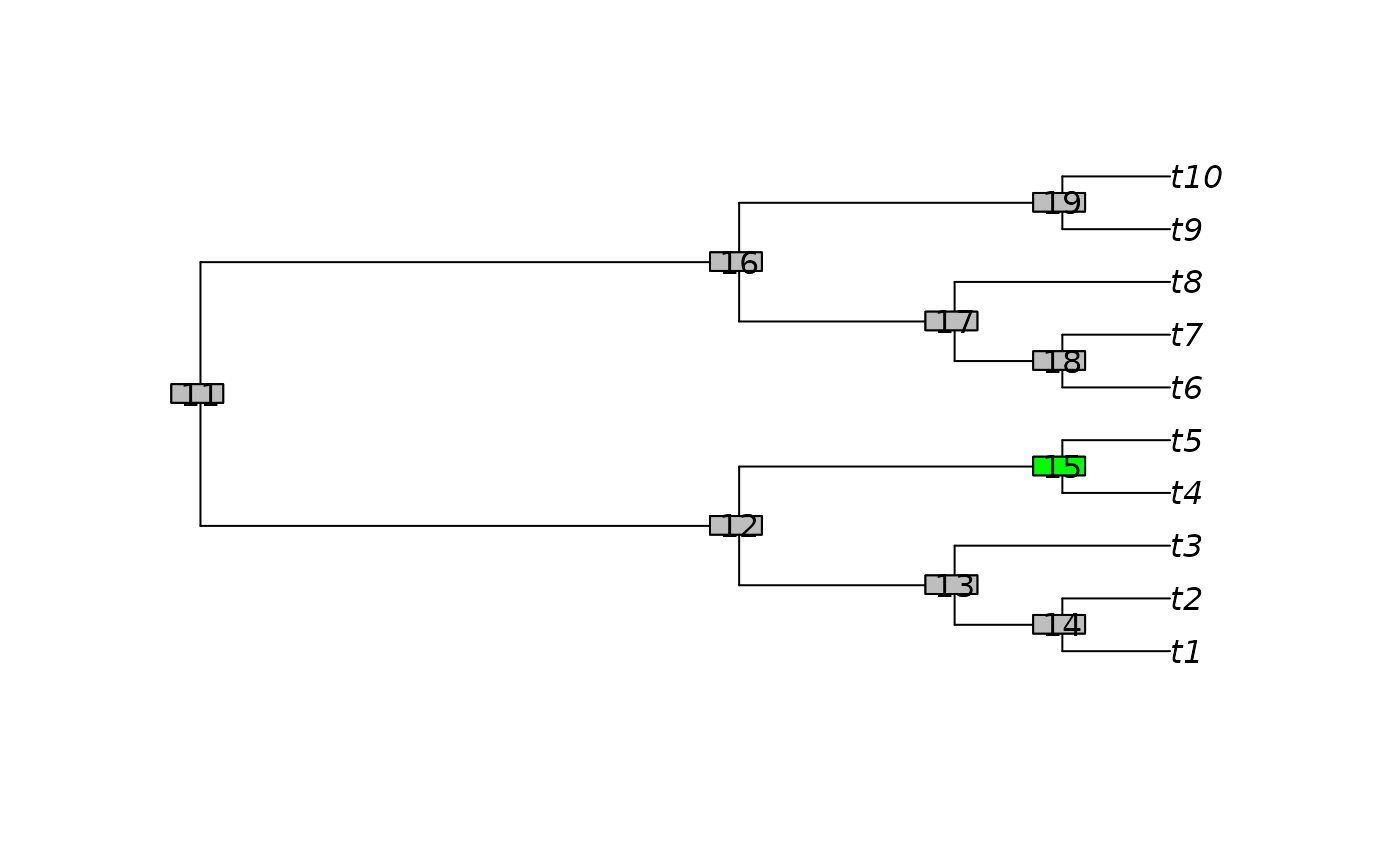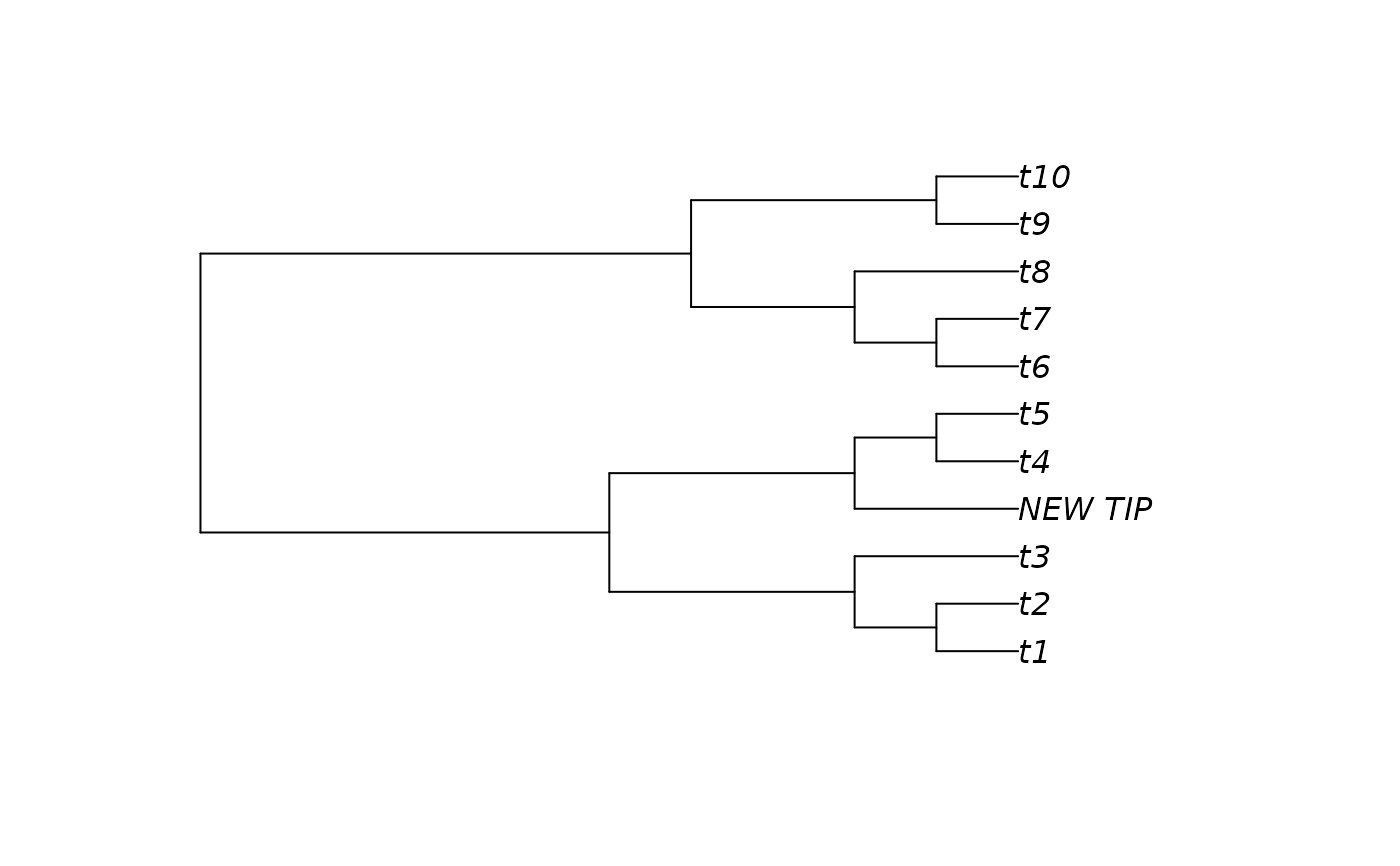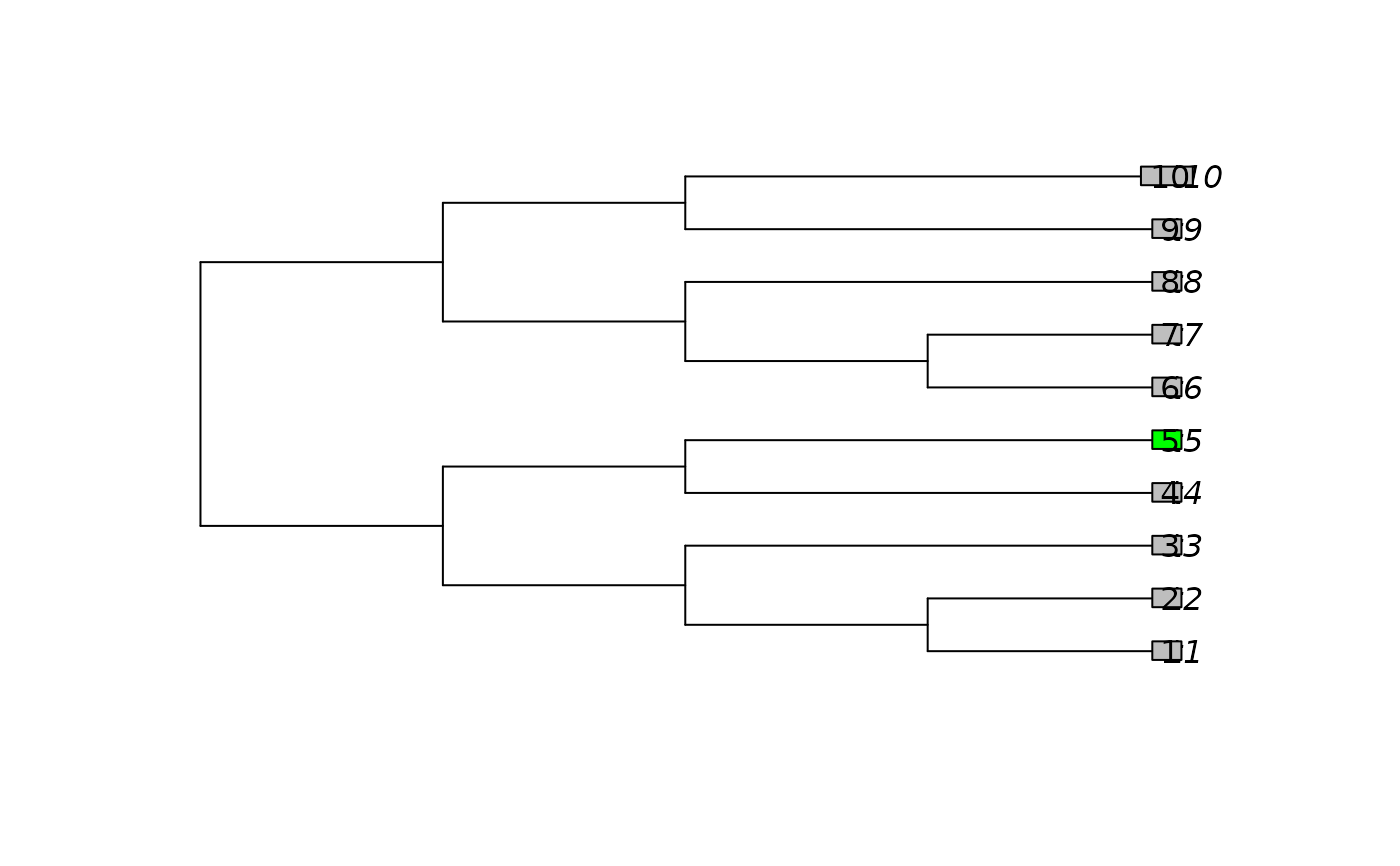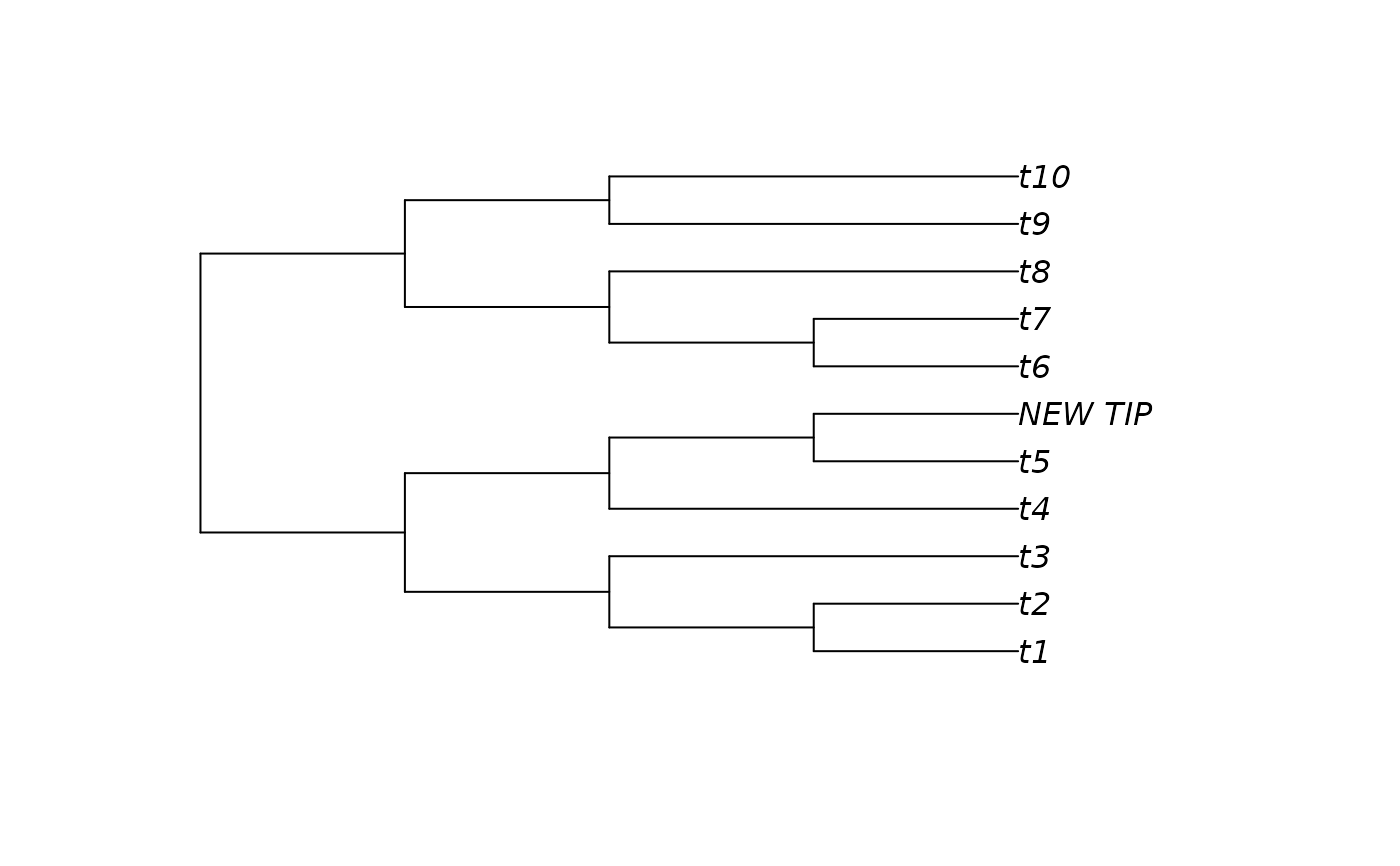AddTip() adds a tip to a phylogenetic tree at a specified location.

AddTip(
tree,
where = sample.int(tree[["Nnode"]] * 2 + 2L, size = 1) - 1L,
label = "New tip",
edgeLength = 0,
lengthBelow = NULL,
nTip = NTip(tree),
nNode = tree[["Nnode"]],
rootNode = RootNode(tree)
)

AddTipEverywhere(tree, label = "New tip", includeRoot = FALSE)

## Arguments

tree

A tree of class phylo.

where

The node or tip that should form the sister taxon to the new node. To add a new tip at the root, use where = 0. By default, the new tip is added to a random edge.

label

Character string providing the label to apply to the new tip.

edgeLength

Numeric specifying length of new edge

lengthBelow

Numeric specifying length below neighbour at which to graft new edge. Values greater than the length of the edge will result in negative edge lengths. If NULL, the default, the new tip will be added at the midpoint of the broken edge. If inserting at the root (where = 0), a new edge of length lengthBelow will be inserted.

nTip, nNode, rootNode

Optional integer vectors specifying number of tips and nodes in tree, and index of root node. Not checked for correctness: specifying values here trades code safety for a nominal speed increase.

includeRoot

Logical; if TRUE, each position adjacent to the root edge is considered to represent distinct edges; if FALSE, they are treated as a single edge.

## Value

AddTip() returns a tree of class phylo with an additional tip at the desired location.

AddTipEverywhere() returns a list of class multiPhylo containing the trees produced by adding label to each edge of tree in turn.

## Details

AddTip() extends bind.tree, which cannot handle single-taxon trees.

AddTipEverywhere() adds a tip to each edge in turn.

Add one tree to another: bind.tree()

Other tree manipulation: CollapseNode(), ConsensusWithout(), DropTip(), EnforceOutgroup(), ImposeConstraint(), KeptPaths(), KeptVerts(), LeafLabelInterchange(), MakeTreeBinary(), RenumberTips(), RenumberTree(), Renumber(), RootTree(), SortTree(), Subtree(), TrivialTree

## Author

Martin R. Smith (martin.smith@durham.ac.uk)

## Examples

plot(tree <- BalancedTree(10))
ape::nodelabels()
ape::nodelabels(15, 15, bg="green")oldPar <- par(mfrow = c(2, 4), mar = rep(0.3, 4), cex = 0.9)

backbone <- BalancedTree(4)
# Treating the position of the root as instructive: